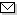Language:   Search:   Contact# Search and Browse the MSC 2000

Search:
Please note that in the second half of 2009, MSC2010 is deployed in the production of ZMATH and will become official standard in January 2010.

Enter a query and click »Search«...

15-XX Linear and multilinear algebra; matrix theory → ZMATH
15-00 General reference works (handbooks, dictionaries, bibliographies, etc.) → ZMATH
15-01 Instructional exposition (textbooks, tutorial papers, etc.) → ZMATH
15-02 Research exposition (monographs, survey articles) → ZMATH
15-03 Historical (must also be assigned at least one classification number from Section 01) → ZMATH
15-04 Explicit machine computation and programs (not the theory of computation or programming) → ZMATH
15-06 Proceedings, conferences, collections, etc. → ZMATH
15A03 Vector spaces, linear dependence, rank → ZMATH
15A04 Linear transformations, semilinear transformations → ZMATH
15A06 Linear equations → ZMATH
15A09 Matrix inversion, generalized inverses → ZMATH
15A15 Determinants, permanents, other special matrix functions [See also 19B10, 19B14] → ZMATH
15A18 Eigenvalues, singular values, and eigenvectors → ZMATH
15A21 Canonical forms, reductions, classification → ZMATH
15A23 Factorization of matrices → ZMATH
15A24 Matrix equations and identities → ZMATH
15A27 Commutativity → ZMATH
15A29 Inverse problems → ZMATH
15A30 Algebraic systems of matrices [See also 16S50, 20Gxx, 20Hxx] → ZMATH
15A33 Matrices over special rings (quaternions, finite fields, etc.) → ZMATH
15A39 Linear inequalities → ZMATH
15A42 Inequalities involving eigenvalues and eigenvectors → ZMATH
15A45 Miscellaneous inequalities involving matrices → ZMATH
15A48 Positive matrices and their generalizations; cones of matrices → ZMATH
15A51 Stochastic matrices → ZMATH
15A52 Random matrices → ZMATH
15A54 Matrices over function rings in one or more variables → ZMATH
15A57 Other types of matrices (Hermitian, skew-Hermitian, etc.) → ZMATH
15A60 Norms of matrices, numerical range, applications of functional analysis to matrix theory [See also 65F35, 65J05] → ZMATH
15A63 Quadratic and bilinear forms, inner products [See mainly 11Exx] → ZMATH
15A66 Clifford algebras, spinors → ZMATH
15A69 Multilinear algebra, tensor products → ZMATH
15A72 Vector and tensor algebra, theory of invariants [See also 13A50, 14L24] → ZMATH
15A75 Exterior algebra, Grassmann algebras → ZMATH
15A78 Other algebras built from modules → ZMATH
15A90 Applications of matrix theory to physics → ZMATH
15A99 Miscellaneous topics → ZMATH

NewsAbel prize 2010I. M. Gelfand 1913-2009MSC2010

Copyright © 2020 Zentralblatt MATH | European Mathematical Society | FIZ Karlsruhe | Heidelberg Academy of Sciences